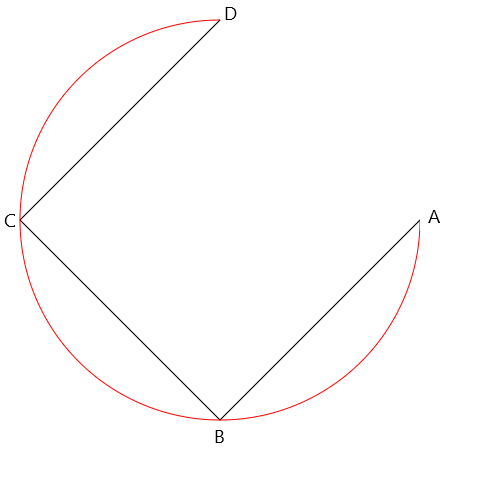# What color are the spinning squares?

Look at these blue spinning squares! I mean black. I mean blue.
Are they blue or black? The answer is: yes.

It's a very simple optical illusion: first, black squares are spinning against a blue background. Then, it's the opposite.
The switch happens when the background and the foreground consist of squares of identical sizes, so your brain tends not to notice the transitions.
It thinks it's one continuous rotation, because in the last 100,000 years of evolution none of our ancestors spent a lot of time watching this type of animation.

Let's take a closer look at the short (25 lines) JavaScript snippet that fools our brains so efficiently. I'm using the JavaScript nomenclature for coordinates and angles.

[1-5] Our HTML page will only consist of a small Canvas.
 The 'degrees' variable will determine the angle of rotation of the squares. Start at zero.
 A small array of color names.
 Parameters of the squares.
 The 'phase' determines whether black squares are spinning on a blue background (if the value is 'true') or the other way round (if 'false').
 Kick off the animation.

[12-31] The 'animate' function is where all the action happens:
 Convert degrees to radians, because the Math.sin takes input in radians.
[14-15] Draw the background. The 'true/false' value is first reversed (so that the background color is the opposite of the squares color) and then converted into 0 and 1 and the respective color is pulled from the 'color' array.
 The squares color is determined similarly, but without the reversal.
[17-18] Draw the squares in rows and columns.
[19-20] Calculate the center of each square:
The squares will rotate in a circle, so the distance between the centers of the squares must be equal to twice the radius, so that each vertex touches another one when the rotation angle equals to multiples of 90 degrees). In other words, the diameter (2*radius) of the circle is the diagonal of the square.
If the phase is 'true', shift the centers by one radius to the right and down, so that the centers of the black squares are placed correctly.
[22-25] Draw the four sides of the square.
We're using this basic formula to find the coordinates of a point on a circle on the Cartesian plane:The first vertex (A) uses the 'angle' rotation. Every subsequent vertex (B, C, D) is rotated by an additional 90 degrees (π/2).
Only draw three sides and then  fill the shape, so the resulting shape is a square. The red arc below shows the circular path from 0 to 270 degrees.
 Increase the rotation angle.
 Reverse the phase every 180 degrees.
 Wait for the next animation frame.

Here is the full code of the editor:



Check out these programming tutorials:

JavaScript:

Optical illusion (18 lines)

Oldschool fire effect (20 lines)

Fireworks (60 lines)

Animated fractal (32 lines)

Physics engine for beginners

Starfield (21 lines)

Yin Yang with a twist (4 circles and 20 lines)

Tile map editor (70 lines)

Sine scroller (30 lines)

Interactive animated sprites

Your first program in JavaScript: you need 5 minutes and a notepad

Fractals in Excel

Python in Blender 3d:Domino effect (10 lines)Wrecking ball effect (14 lines)

3d fractal in Blender Python